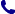• Kontakt
• Newsletter Zapisz się po informacje o nowościach i specjalnych ofertach. Administrator danych: ABE-IPS sp. z o.o.
Wybierz interesujące Cię kategorie
Wyrażam zgodę na przetwarzanie moich danych osobowych w celach marketingowych, tym samym akceptuję Regulamin Newslettera i Politykę prywatności.
Wyrażam zgodę na przesyłanie informacji handlowych drogą elektroniczną.
Wypisz się
• Klienci instytucjonalni
• Dostawa
• O nas
Dostęp on-line

# Książki

0.00 PLN
Schowek (0)
 Schowek jest pusty

## Oxford IB Diploma Programme: IB Mathematics: analysis and approaches, Standard Level, Print and Enhanced Online Course Book Pack

 Autorzy Wydawnictwo Oxford University Press Data wydania 01/02/2019 Wydanie Pierwsze Liczba stron 672 Forma publikacji zestaw Poziom zaawansowania Dla szkół wyższych i kształcenia podyplomowego Język angielski ISBN 9780198427100 Kategorie Matematyka
339.71 PLN (z VAT)
\$92.40 / €75.75 / £65.08 /
Produkt na zamówienie
Dostawa 3-4 tygodnie
Ilość
Do schowka

## Opis książki

Featuring a wealth of digital content, this concept-based Print and Enhanced Online Course Book Pack has been developed in cooperation with the IB to provide the most comprehensive support for the new DP Mathematics: analysis and approaches SL syllabus, for first teaching in September 2019. Each Enhanced Online Course Book Pack is made up of one full-colour, print textbook and one online textbook - packed full of investigations, exercises, worksheets, worked solutions and answers, plus assessment preparation support.

Oxford IB Diploma Programme: IB Mathematics: analysis and approaches, Standard Level, Print and Enhanced Online Course Book Pack

## Spis treści

From patterns to generalizations: sequences and series
1.1: Number patterns and sigma notation
1.2: Arithmetic and geometric sequences
1.3: Arithmetic and geometric series
1.4: Modelling using arithmetic and geometric series
1.5: The binomial theorem
1.6: Proofs
Representing relationships: introducing functions
2.1: What is a function?
2.2: Functional notation
2.3: Drawing graphs of functions
2.4: The domain and range of a function
2.5: Composition of functions
2.6: Inverse functions
Modelling relationships: linear and quadratic functions
3.1: Parameters of a linear function
3.2: Linear functions
3.3: Transformations of functions
3.5: Solving quadratic equations by factorization and completing the square
3.6: The quadratic formula and the discriminant
Equivalent representations: rational functions
4.1: The reciprocal function
4.2: Transforming the reciprocal function
4.3: Rational functions of the form ax+b/cx+d
Measuring change: differentiation
5.1: Limits and convergence
5.2: The derivative function
5.3: Differentiation rules
5.4: Graphical interpretation of first and second derivatives
5.5: Application of differential calculus: optimization and kinematics
Representing data: statistics for univariate data
6.1: Sampling
6.2: Presentation of data
6.3: Measures of central tendency
6.4: Measures of dispersion
Modelling relationships between two data sets: statistics for bivariate data
7.1: Scatter diagrams
7.2: Measuring correlation
7.3: The line of best fit
7.4: Least squares regression
Quantifying randomness: probability
8.1: Theoretical and experimental probability
8.2: Representing probabilities: Venn diagrams and sample spaces
8.3: Independent and dependent events and conditional probability
8.4: Probability tree diagrams
Representing equivalent quantities: exponentials and logarithms
9.1: Exponents
9.2: Logarithms
9.3: Derivatives of exponential functions and the natural logarithmic function
From approximation to generalization: integration
10.1: Antiderivatives and the indefinite integral
10.2: More on indefinite integrals
10.3: Area and definite integrals
10.4: Fundamental theorem of calculus
10.5: Area between two curves
Relationships in space: geometry and trigonometry in 2D and 3D
11.1: The geometry of 3D shapes
11.1: Right-angles triangle trigonometry
11.3: The sine rule
11.4: The cosine rule
11.5: Applications of right and non-right angled trigonometry
Periodic relationships: trigonometric functions801 777 223